## 学个Antenna：偶极子天线馈电及倒V天线

2022-01-11 15:33发布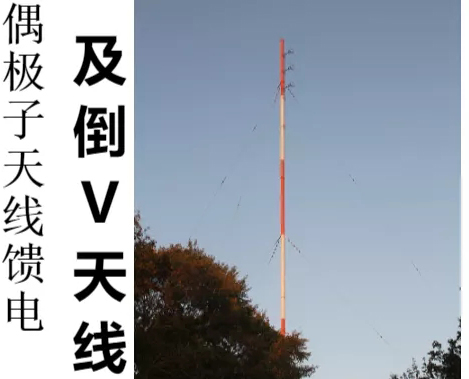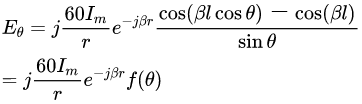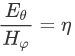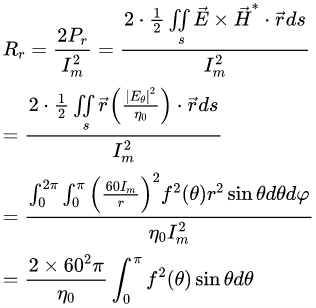%被积函数 L_lambda_array=0:0.01:1;  Rr_array=zeros(1,length(L_lambda_array)); for count=1:length(L_lambda_array)     L_lambda=L_lambda_array(count);    int_term=@(theta)(cos(2*pi*L_lambda*cos(theta)) cos(2*pi*L_lambda)).^2./sin(theta); %计算积分     Rr_array(count)=2*60^2*pi/(120*pi)*integral(@(theta)int_term(theta),0,pi); End plot(L_lambda_array,Rr_array,'b','linewidth',3); xlabel({'${l \mathord{\left/{\vphantom {l \lambda }} \right.\kern \nulldelimiterspace} \lambda }$'},'Interpreter','latex','fontsize',20); ylabel('辐射电阻R_r(\Omega)','fontsize',15); grid on；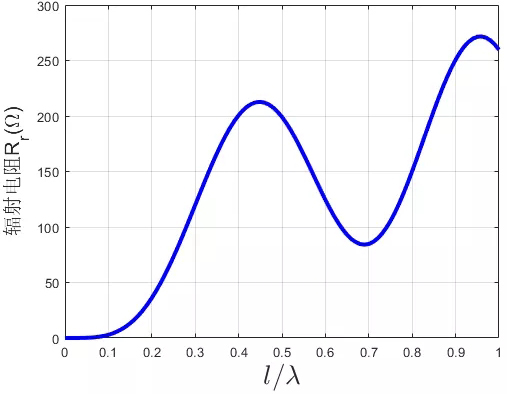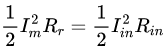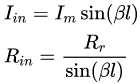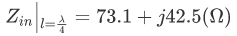坡印亭矢量法: 由远区辐射场求得表示功率密度的坡印亭矢量，然后在以天线中点为圆心，以远区距离为半径的一个球面上积分求得辐射功率，最后求得辐射电阻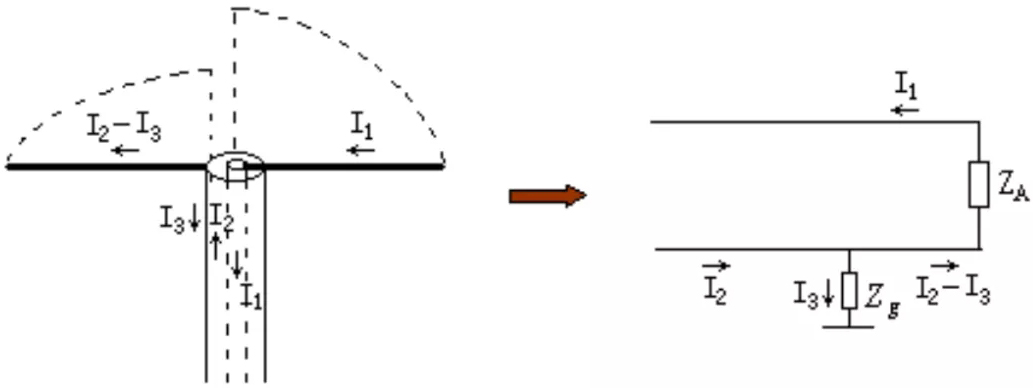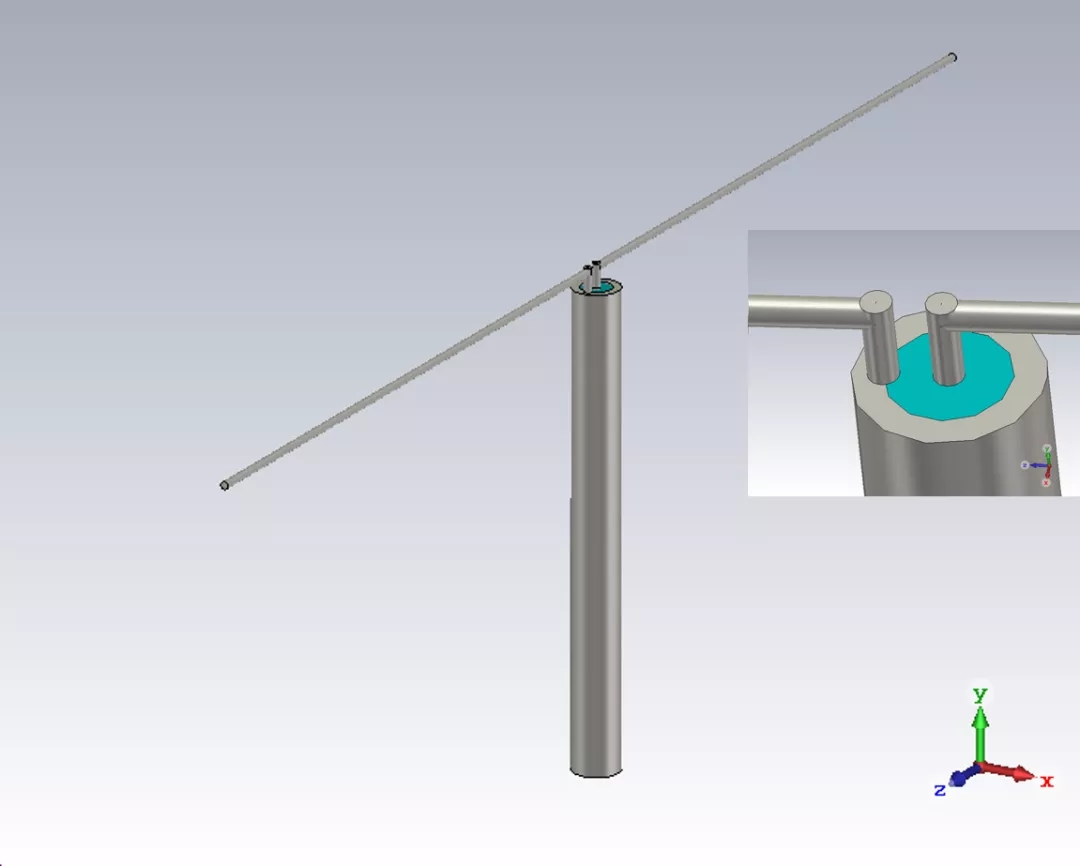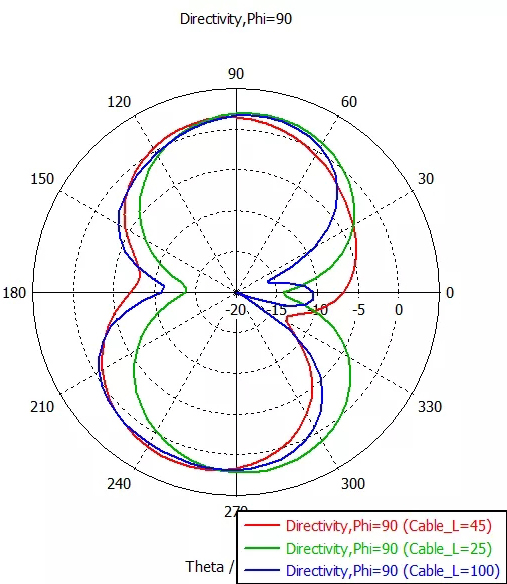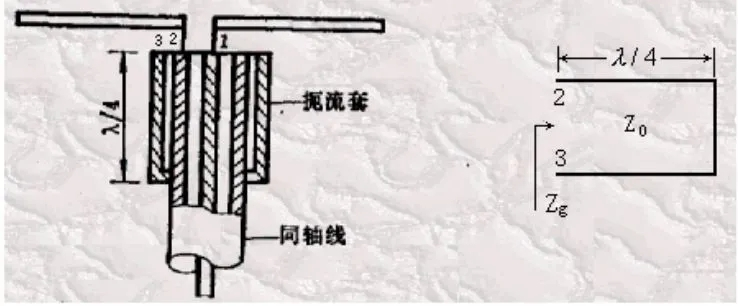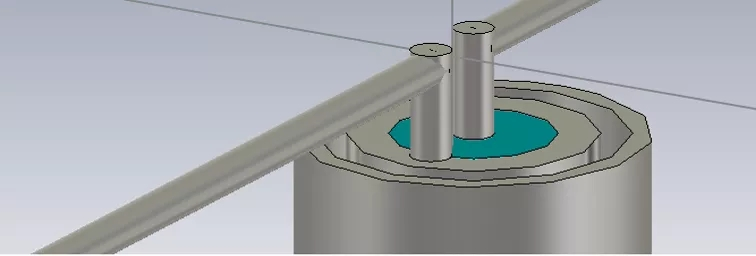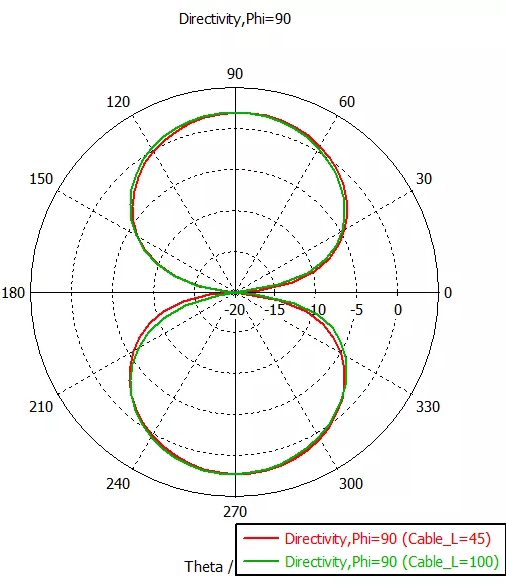HFSS仿真结果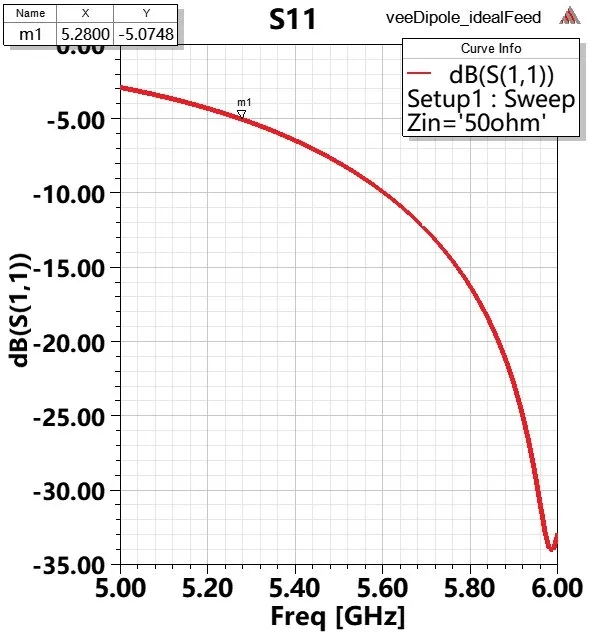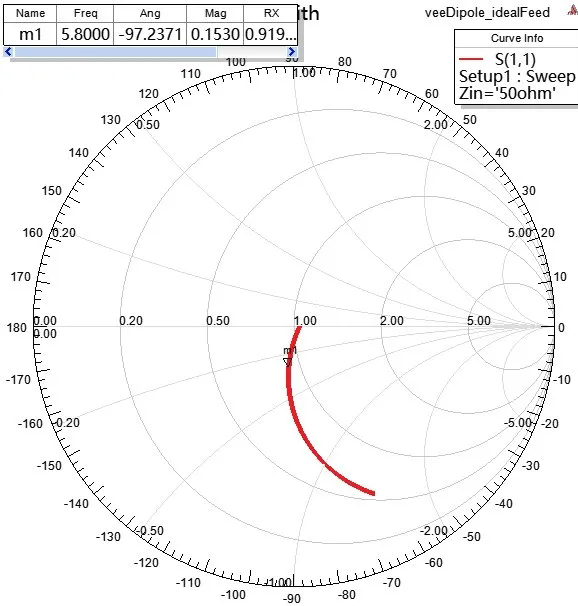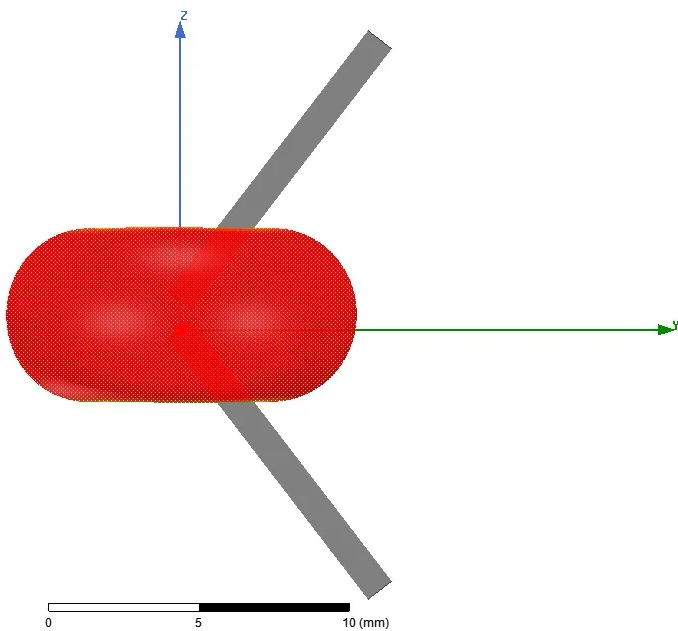http://mouhanei.blog.sohu.com/89131236.html

http://www.51hei.com/bbs/dpj-32878-1.html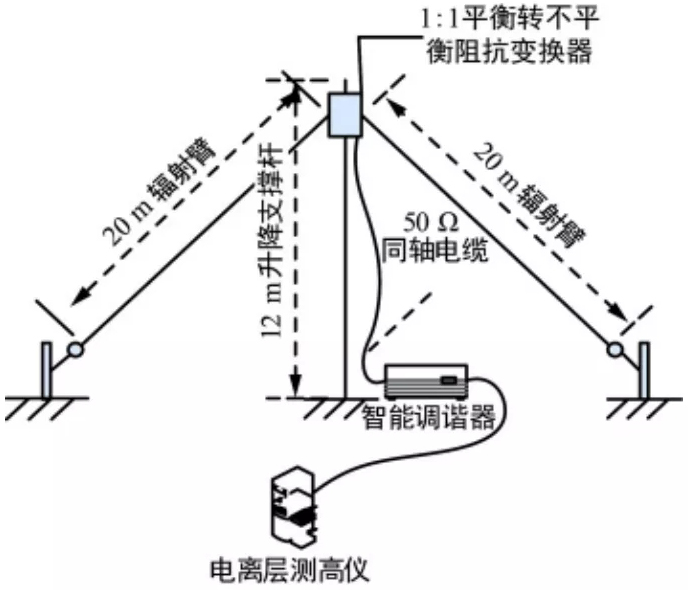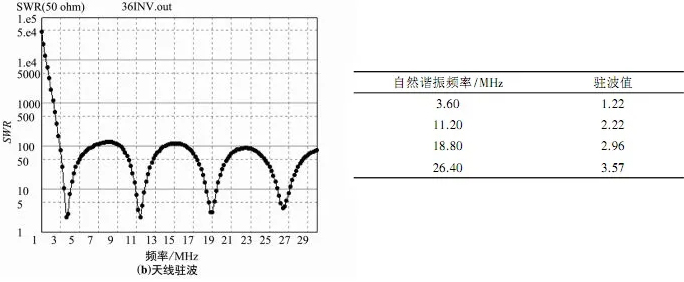HFSS仿真结果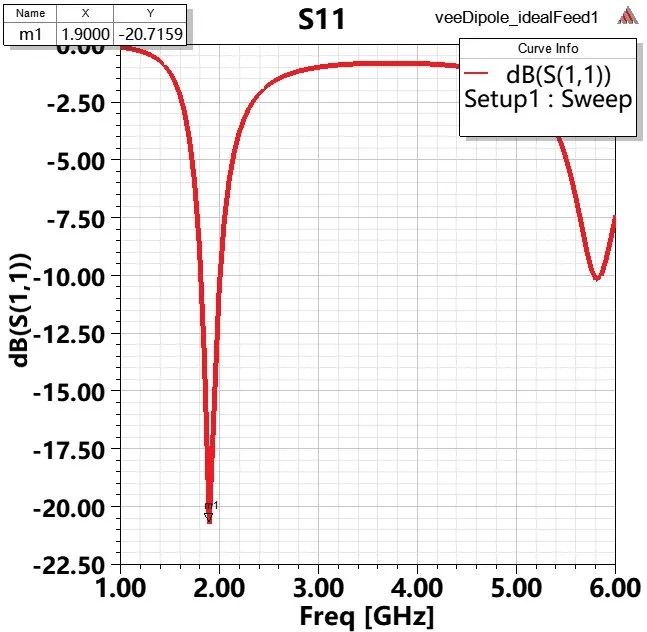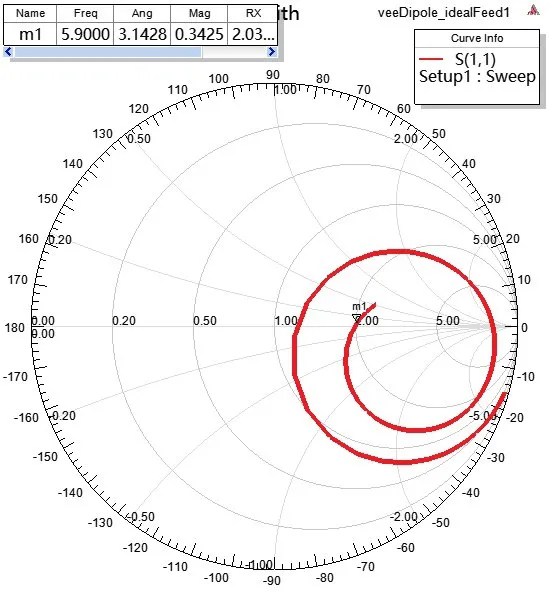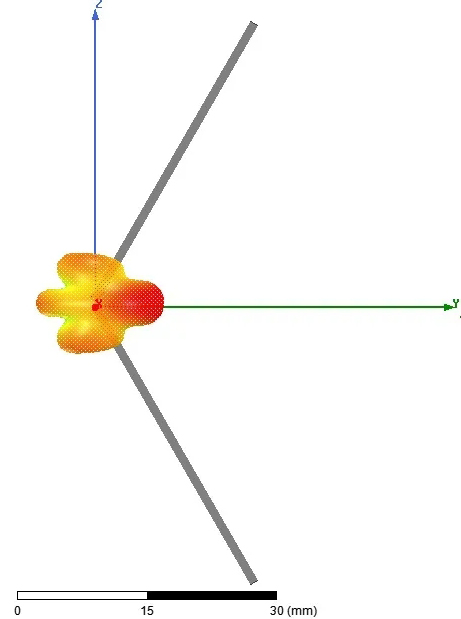便携天馈系统在移动电离层测高中的应用: 秦旭.便携天馈系统在移动电离层测高中的应用[J].无线电通信技术,2017,043(003):71-75.*本文的图片部分来自天线原理相关教材，致谢Markdown Nice提供的公式排版服务

*欢迎左侧一键转发至朋友圈，同时右侧在看与点赞